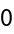#Function Repository Resource:

# FunctionParity

Determine the parity of a function, even or odd, with respect to one or more variables

Contributed by: Wolfram|Alpha Math Team
 ResourceFunction["FunctionParity"][expr,x] gives an integer indicating the parity of expr with respect to x, or Undefined. ResourceFunction["FunctionParity"][expr,{x1,x2,…}] gives the overall parity of expr with respect to multiple variables xi.

## Details and Options

FunctionParity returns one of the following results:
 -1 expr is an odd function of x 1 expr is an even function of x 0 expr is both an even and an odd function of x none of the above is true
A function is considered even if f(x)=f(-x) for all x and is considered odd if -f(x)=f(-x).
For a function of multiple variables, parity is determined by its behavior under the transformation {x,y,..}{-x,-y,..}. It is considered even if it maintains its value under this transformation and odd if it changes sign.

## Examples

### Basic Examples

Find the parity of a basic power function:

 In:=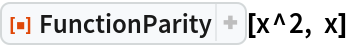Out=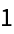Test another power function:

 In:=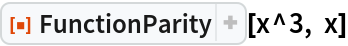Out=Show that a constant function has even parity:

 In:=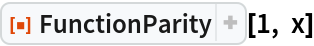Out=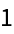Find the parity of a rational function:

 In:=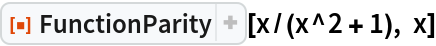Out=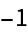Find the parity of the absolute value function:

 In:=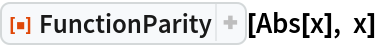Out=Find the parity of a trigonometric function:

 In:=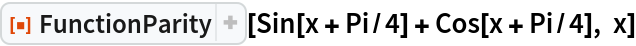Out=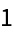Find the parity of a gaussian function:

 In:=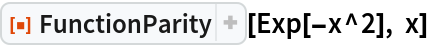Out=Test a signed gaussian function:

 In:=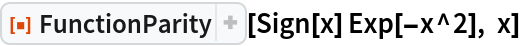Out=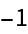Find the parity of the hyperbolic tangent function:

 In:=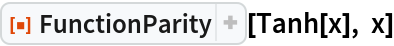Out=Find the parity of the error function:

 In:=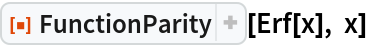Out=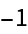Find the parity of a Fresnel integral:

 In:=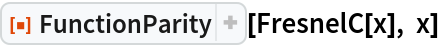Out=Find the parity of a shifted Fresnel integral:

 In:=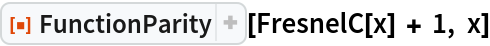Out=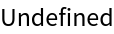### Scope

For a function of multiple variables, parity is determined based on the behavior of the function under the transformation {x,y,..}{-x,-y,..}. Find the parity of a function of two variables:

 In:=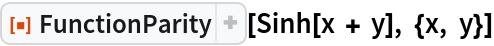Out=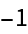Find the parity of a function of three variables:

 In:=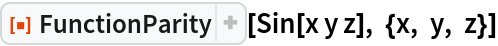Out=Wrapping z in Abs converts this to an even function:

 In:=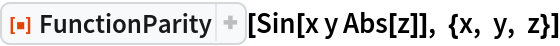Out=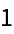Show that an implicitly-defined circle is even in both x and y:

 In:=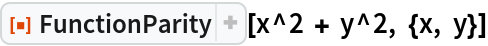Out=### Properties and Relations

FunctionParity returns Undefined for functions that are neither even nor odd:

 In:=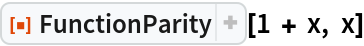Out=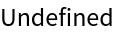The constant function f(x)=0 is both even and odd:

 In:=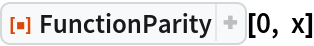Out=